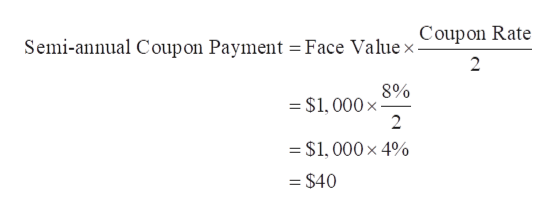# You buy a 20-year bond with a coupon rate of 8.8% that has a yield to maturity of 9.8%. (Assume a face value of \$1,000 and semiannual coupon payments.) Six months later, the yield to maturity is 10.8%. What is your return over the 6 months? (Do not round intermediate calculations. Enter your answer as a percent rounded to 2 decimal places. Negative amount should be indicated by a minus sign.)

Question
29 views

You buy a 20-year bond with a coupon rate of 8.8% that has a yield to maturity of 9.8%. (Assume a face value of \$1,000 and semiannual coupon payments.) Six months later, the yield to maturity is 10.8%. What is your return over the 6 months? (Do not round intermediate calculations. Enter your answer as a percent rounded to 2 decimal places. Negative amount should be indicated by a minus sign.)

check_circle

Step 1

Face Value (FV) = \$1,000

Annual Coupon Rate = 8%

Calculation of semi-annual coupon payment:help_outlineImage TranscriptioncloseSemi-annual Coupon Payment = Face Value x Coupon Rate 2 8% =\$1, 000 x 2 = \$1, 000 x 4% = \$40 fullscreen
Step 2

Face Value (FV) = \$1,000

Annual Coupon Rate = 8%

Semi-annual Coupon Payment (pmt) = \$40

Yield to Maturity (rate) = 9.8%

Time Period = 20 years

Number of payments per year = 2

Time to Maturity (nper) = 40

Calculation of Buying Price of Bond using Excel PV Function:

To open the "PV function" window - MS-Excel --> Formulas --> Financials --> PV.

Step 3

### Want to see the full answer?

See Solution

#### Want to see this answer and more?

Solutions are written by subject experts who are available 24/7. Questions are typically answered within 1 hour.*

See Solution
*Response times may vary by subject and question.
Tagged in